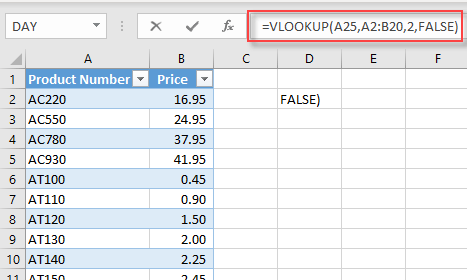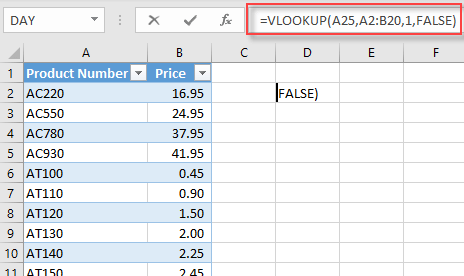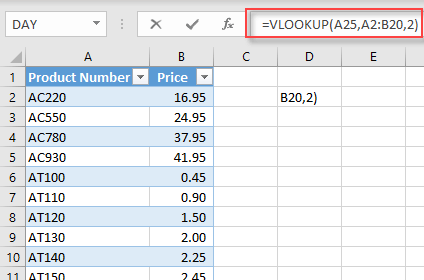Quiz

1. 1
Intermediate

## Suppose cells A2:B20 contain a table with product numbers in column A and product prices in column B. What does the formula =VLOOKUP(A25,A2:B20,2,FALSE) return?2. 2
Intermediate

## Suppose cells A2:B20 contain a table with product numbers in column A and product prices in column B. What does the formula =VLOOKUP(A25,A2:B20,1,FALSE) return?3. 3
Intermediate

## Suppose cells A2:B20 contain a table with sorted product numbers in column A and product prices in column B. What does the formula =VLOOKUP(A25,A2:B20,2) return?